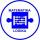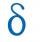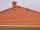# Sequence

Between numbers 1 and 53 insert n members of the arithmetic sequence that its sum is 702.

Result

n =  26

#### Solution:Leave us a comment of example and its solution (i.e. if it is still somewhat unclear...):Be the first to comment!## Next similar examples:

1. AS sequenceIn an arithmetic sequence is given the difference d = -3 and a71 = 455. a) Determine the value of a62 b) Determine the sum of 71 members.
2. Sequence 3Write the first 5 members of an arithmetic sequence: a4=-35, a11=-105.
3. SequenceWrite the first 7 members of an arithmetic sequence: a1=-3, d=6.
4. AS - sequenceWhat are the first ten members of the sequence if a11=22, d=2.
5. SequenceWrite the first 6 members of these sequence: a1 = 5 a2 = 7 an+2 = an+1 +2 an
6. Sequence 2Write the first 5 members of an arithmetic sequence a11=-14, d=-1
7. AP - basicsDetermine first member and differentiate of the the following sequence: a3-a5=24 a4-2a5=61
8. AP - simpleDetermine the first nine elements of sequence if a10 = -1 and d = 4
9. Difference APCalculate the difference of arithmetic progression if the sum of its first 19 members Sn = 8075 and the first member is a1 = 20
10. SeatsSeats in the sport hall are organized so that each subsequent row has five more seats. First has 10 seats. How many seats are: a) in the eighth row b) in the eighteenth row
11. Third memberDetermine the third member of the AP if a4=93, d=7.5.
12. RoofTiles are stacked in rows on the trapezoidal shaped roof. At the ridge is 15 tiles and each subsequent row has one more tile than in the previous row. How many tiled is covered roof if lowermost row has 37 tiles?
13. Cinema 4In cinema are 1656 seats and in the last row are 105 seats , in each next row 3 seats less. How many are the total rows in cinema?
14. Theorem proveWe want to prove the sentence: If the natural number n is divisible by six, then n is divisible by three. From what assumption we started?Find the roots of the quadratic equation: 3x2-4x + (-4) = 0.Solve two equations with two unknowns: 400x+120y=147.2 350x+200y=144Equation ? has one root x1 = 8. Determine the coefficient b and the second root x2.